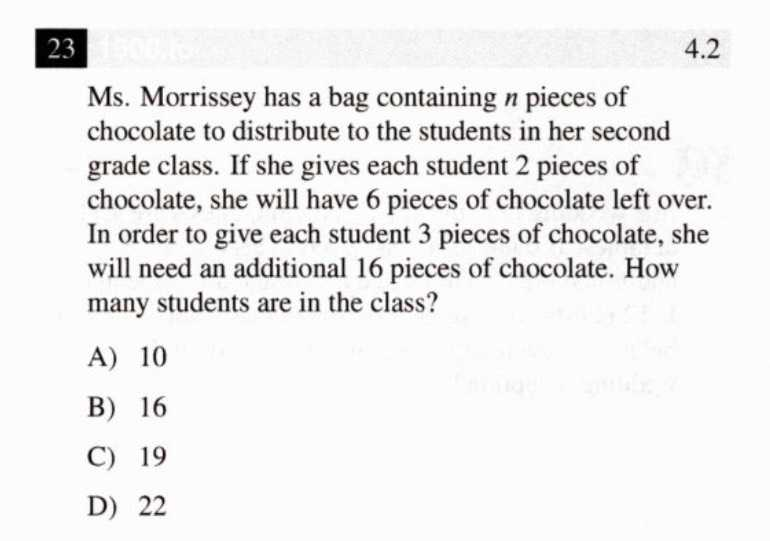### ¿Todavía tienes preguntas de matemáticas?

Pregunte a nuestros tutores expertos
Algebra
Pregunta$$23$$ Ms. Morrissey has a bag containing $$n$$ pieces of chocolate to distribute to the students in her second grade class. If she gives each student $$2$$ pieces of chocolate, she will have $$6$$ pieces of chocolate left over. In order to give each student $$3$$ pieces of chocolate, she will need an additional $$16$$ pieces of chocolate. How many students are in the class? A) $$10$$ B) $$16$$ C) $$19$$ D) $$22$$